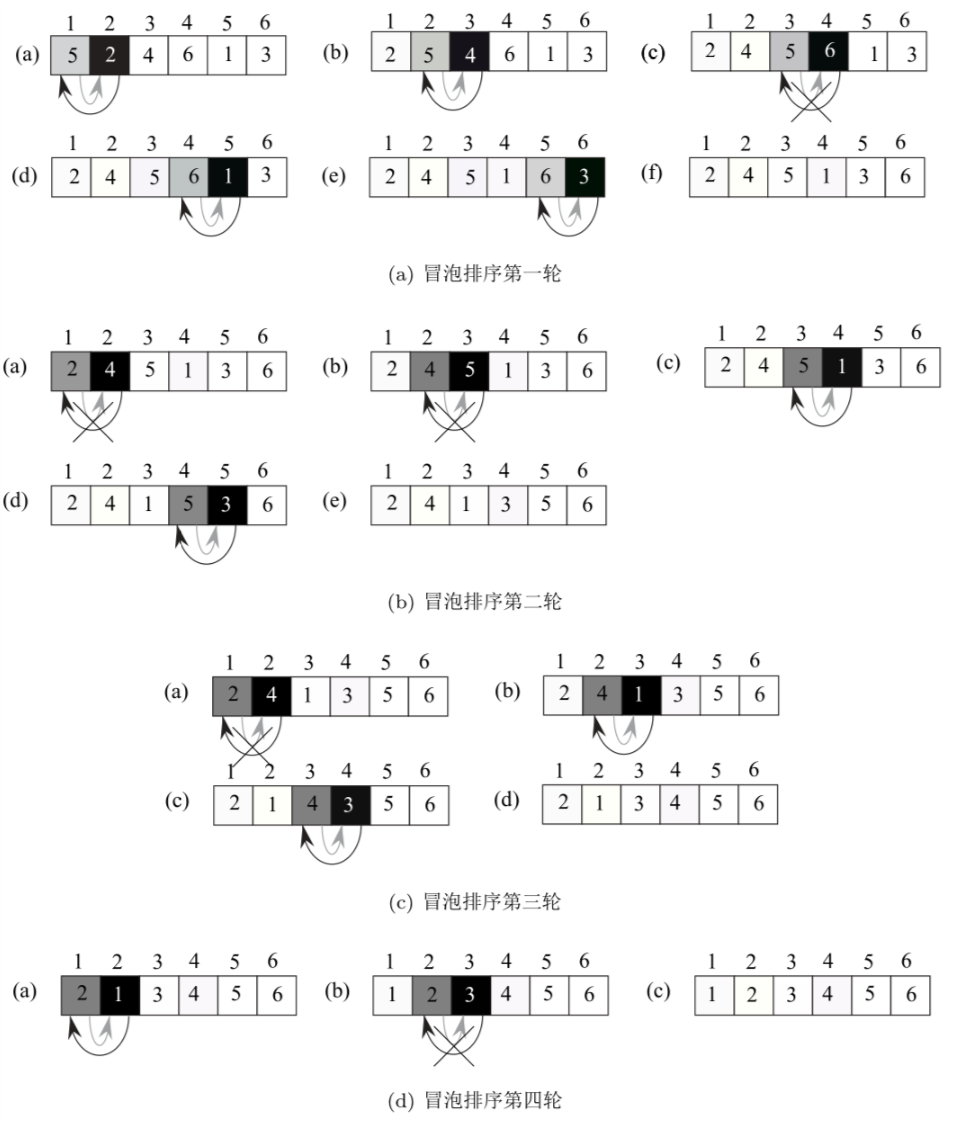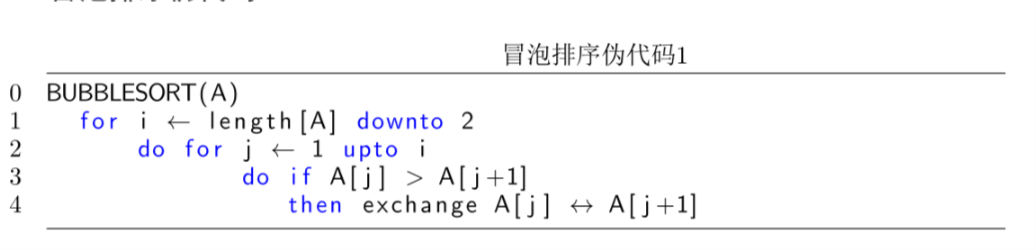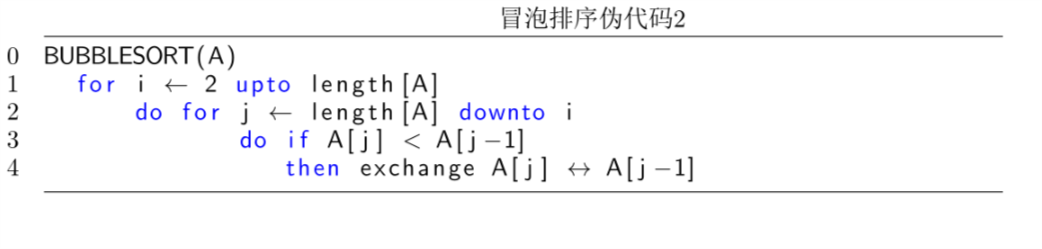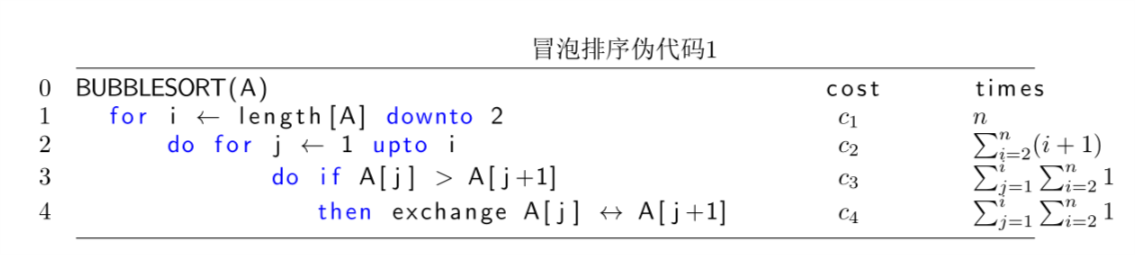# 冒泡排序

## 2 示例Figure 1: $$(5,2,4,6,1,3)$$的冒泡排序过程

## 3 冒泡排序伪代码Figure 2: 冒泡排序伪代码1Figure 3: 冒泡排序伪代码2

## 4 算法分析

1. Initialization 在冒泡法排序伪代码1的第一行，当 $$i=length[A]$$ 时，第一轮循环我们可以得到最大的元素被放在了 $$A[length[A]]$$ 的位置。这是没有问题的。
2. Maintenance 接下来的每一次循环中，$$A[length[A]],A[length[A]-1],A[length[A]-2],\ldots$$里的元素值依次递减。这些值也是数组中除了上次循环找出的最大值之外子数组的最大值。
3. Termination 最后我们验证当循环结束时排序的结果。对于伪代码1中所示的冒泡排序算法来说，当 $$i=2$$时，排序结束。此时内部的 for 循环，实现了 $$A,A,A$$的排序。当$$i=2$$时，内部 for 循环不仅找到了$$A,A,A$$ 中最大的元素，而且还实现了$$A,A$$的排序。 $$i$$没有必要再置为1, 让内部的 for 再排一次 $$A,A$$，因为当 $$i=2$$时，$$A,A$$的排序已经完成了。Figure 4: 冒泡排序伪代码1复杂度

\begin{eqnarray} \label{eq:1} T(n) &=& c_1n + c_2\sum_{i=2}^n(i+1) + c_3\sum_{j=1}^i\sum_{i=2}^{n}1 + c_4\sum_{j=1}^i\sum_{i=2}^{n}1 \\ &=& c_1n + c_2 \frac{(n+4)(n-1)}{2} + c_3(n-1)^2 + c_4(n-1)^2 \end{eqnarray}

## 5 冒泡算法c语言实现

 1: void bubblesort( int A[], int n ){
2:     int i=0,j=0,exchange;
3:     for( i=n;i$>$=2;i$--$ )
4:         {
5:             for(j=0;j<i;j $++$)
6:                 {
7:                     if( A[ j ] $>$ A[ j+1 ])
8:                         {
9:                             exchange = A[ j ];
10:                             A[ j ]   = A[ j+1 ];
11:                             A[j+1 ]  =exchange;
12:                         }
13:                 }
14:         }
15: }